Select Page

# MCQ in English Solutions for CBSE Maths 12 Science Application of Integrals

MCQ in English Solutions for CBSE Maths 12 Science Application of Integrals to enable students to get Solutions in a narrative video format for the specific question.

Expert Teacher provides MCQ Solutions for CBSE Maths 12 Science Application of Integrals through Video Solutions in English language. This video solution will be useful for students to understand how to write an answer in exam in order to score more marks. This teacher uses a narrative style for a question from Application of Integrals not only to explain the proper method of answering question, but deriving right answer too.

Please find the question below and view the Solution in a narrative video format.

Question:

Solution Video in English:

## Similar Questions from CBSE, 12th Science, Maths, Application of Integrals

Question 1 : Find the area enclosed by the parabola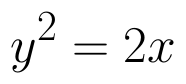and the line x - y = 4. (View Answer Video)

Question 2 : Find the area bounded by the curve y = sin x between x = 0 and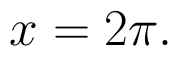(View Answer Video)

Question 3 : Using integration, find the area of the triangle formed by a positive x-axis and tangent and normal to the circle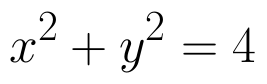at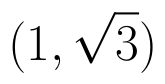. (View Answer Video)

Question 4 : Using integration, find the area of the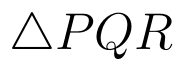co-ordinates whose vertices are P(2,0), Q(4, 5) and R(6,3). (View Answer Video)

Question 5 : Using integration, find the area bounded by the tangent to the curve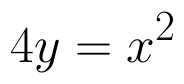at the point (2, 1) and the lines whose equations are x = 2y and x = 3y - 3. (View Answer Video)

### Inverse Trigonometric Functions

Question 1 : Write the principal value of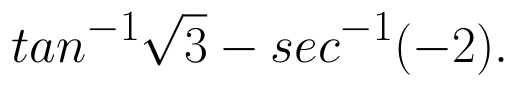(View Answer Video)

Question 2 : Solve the equation for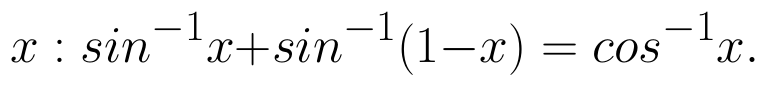(View Answer Video)

Question 3 : Solve for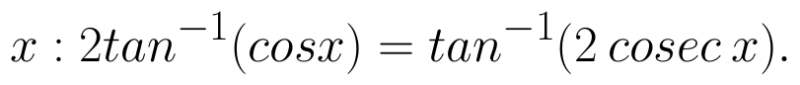(View Answer Video)

Question 4 : Write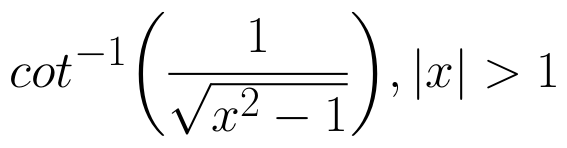in the simplest form. (View Answer Video)

Question 5 : Write in the simplest form: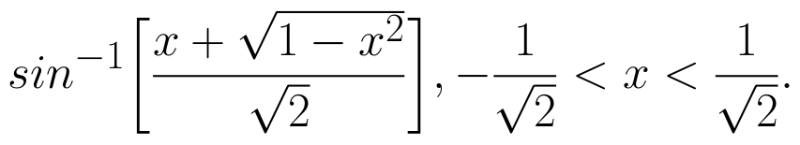(View Answer Video)

### Application of Derivatives

Question 1 : Find approximate value of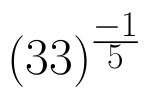. (View Answer Video)

Question 2 : The slope of the tangent to the curve: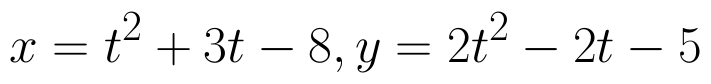Question 3 : Find two numbers whose sum is 24 and whose product is as large as possible. (View Answer Video)

Question 4 : It is given that  at x=1, the function attains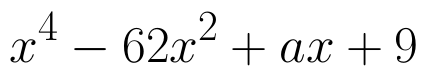its maximum value on the interval[0,2]. Find the value of a? (View Answer Video)

Question 5 : The slope of the tangent to the curve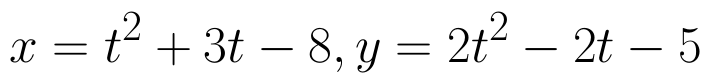at the point (2,-1) is _______________. (View Answer Video)

### Integrals

Question 1 : Write the value of: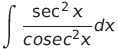(View Answer Video)

Question 2 : Find the integral of the function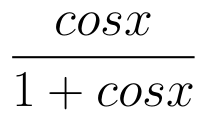. (View Answer Video)

Question 3 : Evaluate :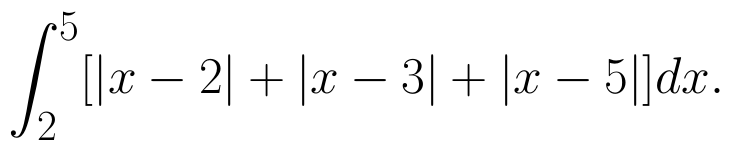(View Answer Video)

Question 4 : Evaluate: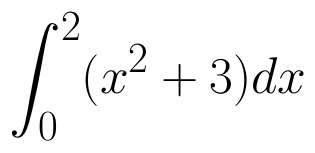as limit of sums.  (View Answer Video)

Question 5 : Find :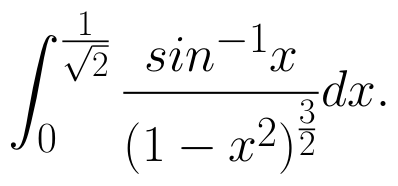(View Answer Video)